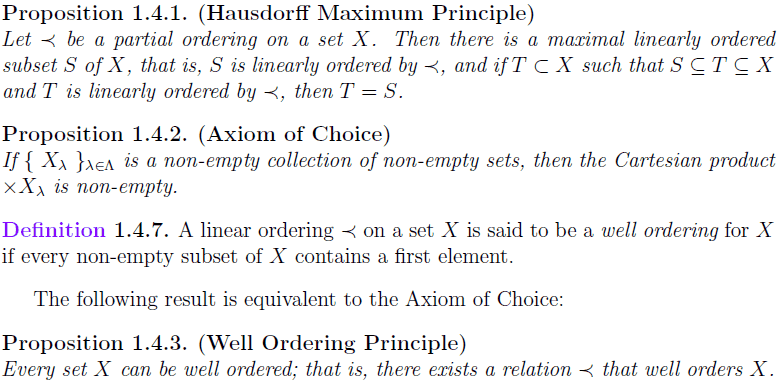# Prove Hausdorff's Maximality Principle by the W.O.P.

• Terrell

## Homework Statement

Show Hausdorff's Maximality Principle is true by the Well-Ordering Principle.

2. Relevant propositions/axioms## The Attempt at a Solution

Case 1: ##\forall x,y\in X## neither ##x\prec y## or ##y\prec x## is true. Hence any singleton subset of ##X## is a maximal linear order.

Case 2: ##\prec## is a linear order on ##X##, then ##X## is itself the maximal linearly ordered subset of ##X##.

Case 3: ##\prec## is undefined on some pairs ##a,b\in X##. Then ##\prec## is not the relation that well-orders ##X## since there exist subsets of ##X## that does not have a least element.
Question: How can I use the Well-Ordering Principle here? I was thinking of defining a new relation ##\prec_w## that well-orders a finite ##W\subseteq X##, but I'm thinking that that cannot be right since ##\prec_w## is not an arbitrary partial-order relation in ##X##. Which part of this problem should I think more about in order to help me proceed? Or May I simply say that we remove all elements in ##X## that has an undefined relation with all other elements in ##X##?

EDIT: (Alternative case 3: W.O.P.##\rightarrow## A.C. ##\rightarrow## H.M.P.)
Suppose ##\{X_{\lambda}\}_{\lambda\in\Lambda}\neq\emptyset## and ##\forall\lambda\in\Lambda, X_{\lambda}\neq\emptyset##. We want to show ##\prod_{\lambda\in\Lambda}X_{\lambda}\neq\emptyset##. By the well-ordering principle, ##\exists\prec_{x}\forall\lambda\in\Lambda##, ##X_\lambda## is well-ordered; that is with respect to ##\prec_x##, ##\forall\lambda\in\Lambda\forall W\subseteq X_{\lambda}##, ##W## has a least element. Hence, we can simply define a choice-function ##f: \{X_\lambda\}_{\lambda\in\Lambda}\rightarrow\bigcup_{\lambda\in\Lambda}X_\lambda## such that ##f(X_{\lambda})=x_{0}^{\lambda}## denote the least element in ##X_{\lambda}##. Therefore, ##\prod_{\lambda\in\Lambda}X_{\lambda}\neq\emptyset##.

Finally, we want to show A.C. ##\rightarrow## H.M.P. Suppose ##\prec## does not well-order ##X##, then ##\exists a,b\in X## where neither ##a\prec b## or ##b\prec a## are true. Consider some partition of ##X## denoted ##P[X]##. Define ##\hat{X}:=\{\hat{x}\in X:\forall\overline{X}\in P[X]\forall x\in\overline{X},\neg (\hat{x}\prec x\quad\lor\quad x\prec\hat{x})\}##. By the axiom of choice, ##\hat{X}\neq\emptyset##. Thus, ##X^*=X\setminus\hat{X}## is linearly ordered such that ##\forall x\in\hat{X}, X^*\cup\{x\}## is no longer linearly ordered. Therefore, ##X^*\subseteq X## is a maximal linearly ordered subset of ##X##.

So I think I have two questions. First, is how to proceed directly from W.O.P. to H.M.P. and second, is I would like a verification on my alternative case 3 (i.e. did I use W.O.P. and A.C. correctly?)

#### Attachments

Last edited:

•